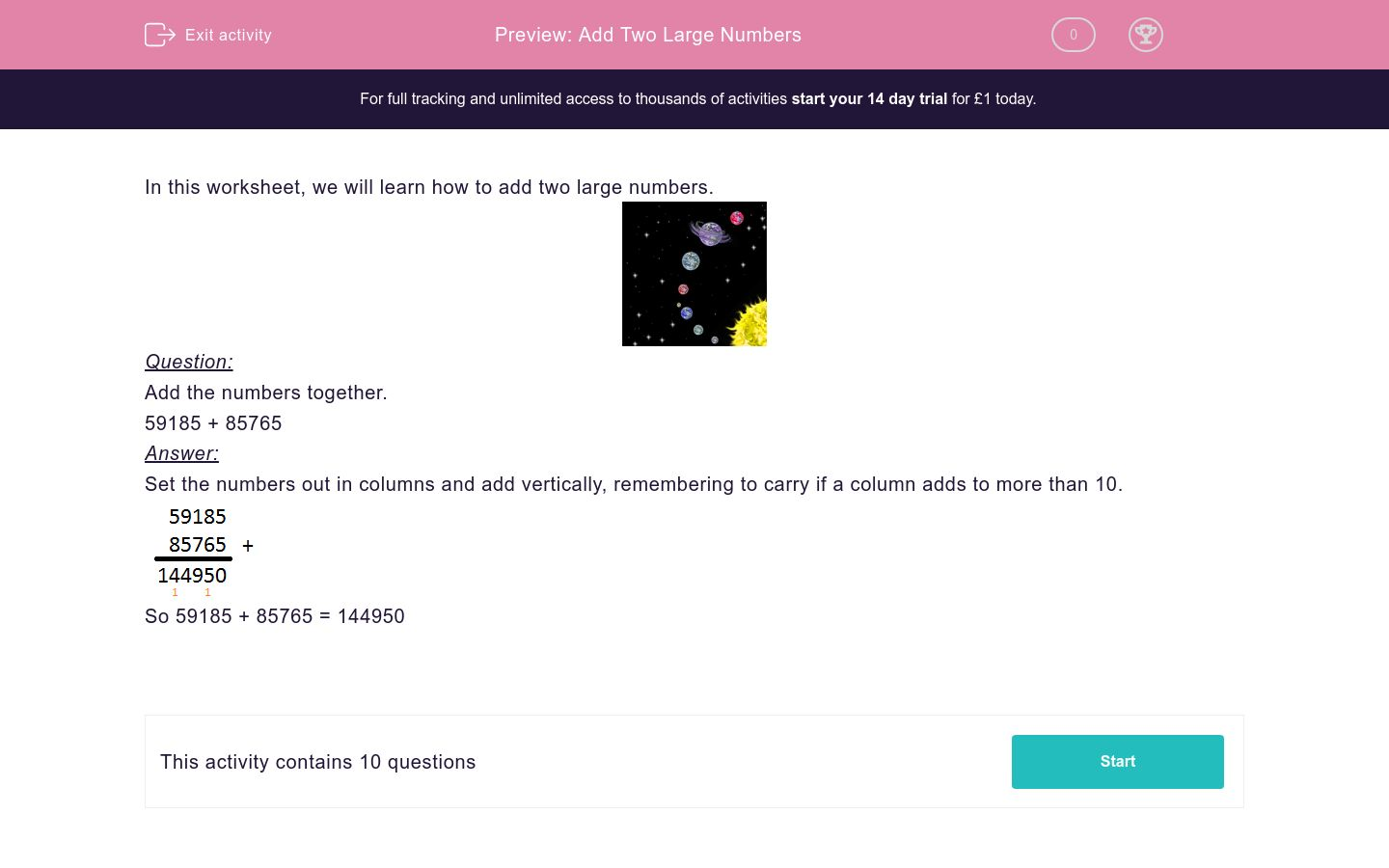### Affordable pricing from £10/month

In this worksheet, students use the column method to add together large numbers resulting in 5-digit and 6-digit answers.Key stage:  KS 2

Curriculum topic:   Maths and Numerical Reasoning

Curriculum subtopic:   Mixed Problems

Difficulty level:### QUESTION 1 of 10

In this worksheet, we will learn how to add two large numbers.Question:

59185 + 85765

Set the numbers out in columns and add vertically, remembering to carry if a column adds to more than 10.So 59185 + 85765 = 144950

92270 + 63096  =  ____________

38032 + 22243  =  ____________

78542 + 58532  =  ____________

84906 + 44004  =  ____________

23746 + 57725  =  ____________

85502 + 76805  =  ____________

19791 + 23533  =  ____________

19791 + 23533  =  ____________

66243 + 46338  =  ____________

19263 + 49552  =  ____________

• Question 1

92270 + 63096  =  ____________

155366
• Question 2

38032 + 22243  =  ____________

60275
• Question 3

78542 + 58532  =  ____________

137074
• Question 4

84906 + 44004  =  ____________

128910
• Question 5

23746 + 57725  =  ____________

81471
• Question 6

85502 + 76805  =  ____________

162307
• Question 7

19791 + 23533  =  ____________

43324
• Question 8

19791 + 23533  =  ____________

43324
• Question 9

66243 + 46338  =  ____________

112581
• Question 10

19263 + 49552  =  ____________

68815
---- OR ----

Sign up for a £1 trial so you can track and measure your child's progress on this activity.

### What is EdPlace?

We're your National Curriculum aligned online education content provider helping each child succeed in English, maths and science from year 1 to GCSE. With an EdPlace account you’ll be able to track and measure progress, helping each child achieve their best. We build confidence and attainment by personalising each child’s learning at a level that suits them.

Get started